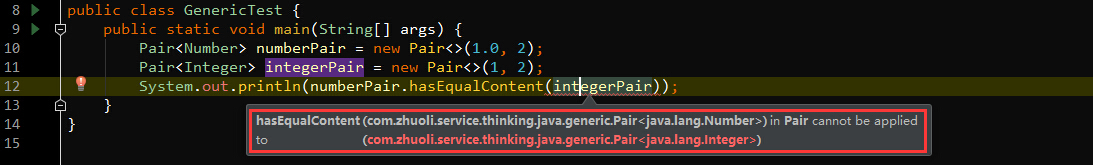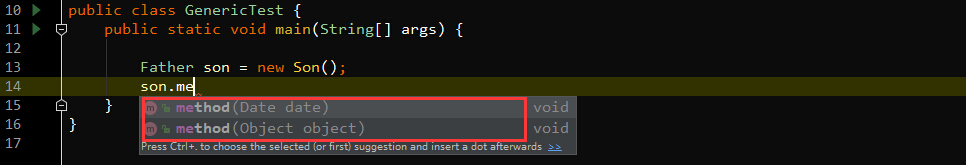coding……

# Java编程拾遗『泛型——基本概念』

## 1. 为什么要使用泛型程序设计？

“泛型”的意思就是广泛的类型，类、接口和方法代码不再与具体类型绑定在一起，可以应用于非常广泛的类型，同一套代码，可以用于多种数据类型。在Java中增加泛型类之前，泛型程序设计是用继承实现的，比如ArrayList类就维护了一个Object引用的数组：

``````//before generic classes
public class ArrayList {

private Object[] elementData;
……

public Object get(int i) {
……
}

……
}
}``````

``````ArrayList files = new ArrayList();
String fileName = (String) files.get(0);``````

``files.add(new File("……"));``

``ArrayList<String> files = new ArrayList<String>();``

``ArrayList<String> files = new ArrayList<>();``

## 2. 泛型类

``````public class Pair<T> {

private T first;
private T second;

public Pair() {

}

public Pair(T first, T second) {
this.first = first;
this.second = second;
}

public T getFirst() {
return first;
}

public T getSecond() {
return second;
}

public void setFirst(T first) {
this.first = first;
}

public void setSecond(T second) {
this.second = second;
}
}``````

``````public class Pair<T, U> {

private T first;
private U second;

……
}``````

``````//成员变量
private String first;
private String second;

//构造函数
public Pair()
public Pair(String first, String second)

//getter and setter
public String getFirst()
public String getSecond()
public void setFirst(String first)
public void setSecond(String second)``````

``````public class ArrayAlg {

public static Pair<String> minMax(String[] array) {
if (array == null || array.length == 0) {
return null;
}

String min = array;
String max = array;
for (int i = 1; i < array.length; i++) {
if (min.compareTo(array[i]) > 0) {
min = array[i];
}

if (max.compareTo(array[i]) < 0) {
max = array[i];
}
}
return new Pair<>(min, max);
}

}``````

## 3. 泛型方法

``````public static <T> T getMiddle(T[] array) {
if (array == null || array.length == 0) {
return null;
}

return array[array.length / 2];
}``````

``````String[] array = new String[]{"A", "B", "C", "D"};
String middle = ArrayAlg.<String>getMiddle(array);``````

``````String[] array = new String[]{"A", "B", "C", "D"};
String middle = ArrayAlg.getMiddle(array);``````

``````public static <T extends Comparable<T>> Pair<T> minMax(T[] array) {
if (array == null || array.length == 0) {
return null;
}

T min = array;
T max = array;
for (int i = 1; i < array.length; i++) {
if (min.compareTo(array[i]) > 0) {
min = array[i];
}

if (max.compareTo(array[i]) < 0) {
max = array[i];
}
}
return new Pair<>(min, max);
}``````

## 4. 泛型接口

Java中，接口也可以是泛型的，比如Java API中的Comparable和Comparator接口都是泛型接口，如下：

``````public interface Comparable<T> {
public int compareTo(T o);
}
public interface Comparator<T> {
int compare(T o1, T o2);
boolean equals(Object obj);
}``````

``````public final class Integer extends Number implements Comparable<Integer>{
public int compareTo(Integer anotherInteger) {
return compare(this.value, anotherInteger.value);
}
//...
}``````

## 5. 类型变量的限定

### 5.1 上界是具体类

``````public class NumberPair<U extends Number> extends Pair<U> {

public NumberPair(U first, U second) {
super(first, second);
}
}    ``````

``````public double sum(){
return getFirst().doubleValue() + getSecond().doubleValue();
}``````

``````NumberPair<Integer> pair = new NumberPair<>(1, 2);
double sum = pair.sum();``````

NumberPair泛型类型指定为Integer，如果指定为String就会编译报错，因为String并不是Number的子类。

### 5.2 上界是接口

``````public static <T extends Comparable<T>> Pair<T> minMax(T[] array) {
if (array == null || array.length == 0) {
return null;
}

T min = array;
T max = array;
for (int i = 1; i < array.length; i++) {
if (min.compareTo(array[i]) > 0) {
min = array[i];
}

if (max.compareTo(array[i]) < 0) {
max = array[i];
}
}
return new Pair<>(min, max);
}
``````

<T extends Comparable<T>>也称为 递归类型限制。

### 5.3 上界是类型变量

``````public boolean hasEqualContent(Pair<T> other) {
return new BigDecimal(getFirst().toString()).compareTo(new BigDecimal(other.getFirst().toString())) == 0
&& new BigDecimal(getSecond().toString()).compareTo(new BigDecimal(other.getSecond().toString())) == 0;
}``````

``````Pair<Number> numberPair = new Pair<>(1.0, 2);
Pair<Integer> integerPair = new Pair<>(1, 2);
System.out.println(numberPair.hasEqualContent(integerPair));````````````Pair<Number> numberPair = new Pair<>();
Pair<Integer> integerPair = new Pair<>();

//假如下面这行是合法的
numberPair = integerPair；

/*numberPair引用地址跟IntegerPair引用地址一致

numberPair.setFirst(1.0);``````

``````public <E extends T> boolean hasEqualContent(Pair<E> other) {
return new BigDecimal(getFirst().toString()).compareTo(new BigDecimal(other.getFirst().toString())) == 0
&& new BigDecimal(getSecond().toString()).compareTo(new BigDecimal(other.getSecond().toString())) == 0;
}``````

T是泛型类Pair的类型变量，E是泛型方法hasEqualContent的类型变量，E的上界是T，这样使用时就没有问题了，如下：

``````public class GenericTest {
public static void main(String[] args) {
Pair<Number> numberPair = new Pair<>(1.0, 2);
Pair<Integer> integerPair = new Pair<>(1, 2);
System.out.println(numberPair.hasEqualContent(integerPair));
}
}``````

``true``

## 6. 泛型代码和虚拟机

Java泛型类只是对于Java编译器的概念，Java编译器会把泛型代码转换为普通的非泛型代码，对于Java虚拟机而言，所有的对象都属于普通类，Java虚拟机是不会感知类型变量的存在的。所有的泛型类型，对于Java虚拟机来讲都有一个相应的原始类型，原始类型相当于泛型类型删掉类型参数，擦除类型变量并替换为限定类型(无限定的类型变量替换为Object)之后的形式。比如泛型类Pair<T>的原始类型如下：

``````public class Pair {

private Object first;
private Object second;

public Pair() {

}

public Pair(Object first, Object second) {
this.first = first;
this.second = second;
}

public Object getFirst() {
return first;
}

public Object getSecond() {
return second;
}

public void setFirst(Object first) {
this.first = first;
}

public void setSecond(Object second) {
this.second = second;
}
}``````

``````public class Interval<T extends Comparable<T> & Serializable> implements Serializable {
private T lower;
private T upper;

//……

public Interval(T first, T second) {
if (first.compareTo(second) <=0) {
this.lower = first;
this.upper = second;
} else {
this.lower = second;
this.upper = first;
}
}
}``````

``````public class Interval implements Serializable {
private Comparable lower;
private Comparable upper;

//……

public Interval(Comparable first, Comparable second) {
//……
}
}``````

### 6.1 泛型表达式

``````public T getFirst() {
return this.first;
}``````

``````public Object getFirst() {
return this.first;
}``````

``````Pair<String> stringPair = new Pair<>("this", "is");
String first = stringPair.getFirst();``````

• 对擦除后的原始方法getFirst()调用
• 将返回的Object类型强制转换为String类型

### 6.2 类型擦除与多态(运行时)

Java中，基类的引用变量不仅可以指向基类的实例对象，也可以指向其子类的实例对象。同样，接口的引用变量也可以指向其实现类的实例对象。当使用接口(基类)的引用变量进行方法调用时，并不是直接调用父类的方法，而是在方法运行时，通过引用变量的具体指向，决定具体方法调用(指向子类实例对象，那么调用具体子类的方法；指向父类实例对象就调用父类的方法，如果子类中没有覆盖父类该方法，会调用父类的方法。)。也就是方法在程序运行才动态绑定，因此通过覆盖实现的多态也被称为运行时多态。

``````public class Father {
public void method(Object object) {
System.out.println("Father method, parameter is :" + object);
}
}``````

``````public class Son extends Father {

public void method(Date date) {
System.out.println("Son overload method, parameter is :" + date);
}
}``````

``````public class GenericTest {
public static void main(String[] args) {

Father son = new Son();
son.method(new Date());
}
}``````

``Father method, parameter is :Fri Nov 09 09:54:32 CST 2018``

``````public class DateInterval extends Pair<Date> {
@Override
public void setSecond(Date second) {
if (second.compareTo(getFirst()) >= 0) {
super.setSecond(second);
}
}
}``````

``````public class Pair {

private Object first;
private Object second;

//……

public Object getFirst() {
return first;
}

public Object getSecond() {
return second;
}

public void setFirst(Object first) {
this.first = first;
}

public void setSecond(Object second) {
this.second = second;
}
}``````

``````Pair<Date> dateInterval = new DateInterval();
dateInterval.setSecond(new Date());````````````public void setSecond(Object second) {
setSecond((Date) second);
}``````

``````public class DateInterval extends Pair<Date> {
@Override
public void setSecond(Date second) {
if (second.compareTo(getFirst()) >= 0) {
super.setSecond(second);
}
}

@Override
public Date getSecond() {
return super.getSecond();
}
}````````````public Date getSecond();
public Object getSecond();``````

• Java虚拟机中没有泛型，只有普通类和方法
• 所有的类型参数，都用它们的限定类型替换(没有限定类型替换为Object)
• Java编译器会生成桥方法用于保持多态的特性
• 使用泛型时，并不需要显示强制类型转换，因为这一过程，被Java编译器实现了

1. 《Java核心技术 卷Ⅰ》
2. 《Java编程的逻辑》
3.  java 泛型详解-绝对是对泛型方法讲解最详细的，没有之一
4. Java泛型详解
5. java 泛型的类型擦除与桥方法

• QQ咨询
• 回顶